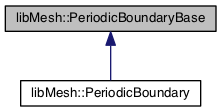libMesh::PeriodicBoundaryBase Class Referenceabstract

Base class for all PeriodicBoundary implementations. More...

`#include <periodic_boundary_base.h>`

Inheritance diagram for libMesh::PeriodicBoundaryBase:[legend]

## Public Types

enum  TransformationType { FORWARD =0, INVERSE =1 }

## Public Member Functions

PeriodicBoundaryBase ()

PeriodicBoundaryBase (const PeriodicBoundaryBase &other)

virtual ~PeriodicBoundaryBase ()

virtual Point get_corresponding_pos (const Point &pt) const =0

virtual std::unique_ptr< PeriodicBoundaryBaseclone (TransformationType t=FORWARD) const =0

void set_variable (unsigned int var)

void merge (const PeriodicBoundaryBase &pb)

bool is_my_variable (unsigned int var_num) const

bool has_transformation_matrix () const

const DenseMatrix< Real > & get_transformation_matrix () const

void set_transformation_matrix (const DenseMatrix< Real > &matrix)

const std::set< unsigned int > & get_variables () const

## Public Attributes

boundary_id_type myboundary

boundary_id_type pairedboundary

## Protected Attributes

std::set< unsigned int > variables

std::unique_ptr< DenseMatrix< Real > > _transformation_matrix

## Detailed Description

Base class for all PeriodicBoundary implementations.

The base class for defining periodic boundaries.

Date
2010

Definition at line 49 of file periodic_boundary_base.h.

## ◆ TransformationType

Enumerator
FORWARD
INVERSE

Definition at line 52 of file periodic_boundary_base.h.

53  { FORWARD=0,
54  INVERSE=1 };

## ◆ PeriodicBoundaryBase() [1/2]

 libMesh::PeriodicBoundaryBase::PeriodicBoundaryBase ( )

Constructor

Definition at line 29 of file periodic_boundary_base.C.

29  :
32 {
33 }
static const boundary_id_type invalid_id

## ◆ PeriodicBoundaryBase() [2/2]

 libMesh::PeriodicBoundaryBase::PeriodicBoundaryBase ( const PeriodicBoundaryBase & other )

Copy constructor

Definition at line 37 of file periodic_boundary_base.C.

References _transformation_matrix.

37  :
38  myboundary(o.myboundary),
39  pairedboundary(o.pairedboundary),
40  variables(o.variables)
41 {
42  // Make a deep copy of _transformation_matrix, if it's not null
43  if(o._transformation_matrix)
44  {
45  this->_transformation_matrix = libmesh_make_unique<DenseMatrix<Real>>();
46  *(this->_transformation_matrix) = *(o._transformation_matrix);
47  }
48 }
std::set< unsigned int > variables
std::unique_ptr< DenseMatrix< Real > > _transformation_matrix

## ◆ ~PeriodicBoundaryBase()

 virtual libMesh::PeriodicBoundaryBase::~PeriodicBoundaryBase ( )
inlinevirtual

Destructor

Definition at line 74 of file periodic_boundary_base.h.

74 {}

## ◆ clone()

 virtual std::unique_ptr libMesh::PeriodicBoundaryBase::clone ( TransformationType t = `FORWARD` ) const
pure virtual

If we want the DofMap to be able to make copies of references and store them in the underlying map, this class must be clone'able, i.e. have a kind of virtual construction mechanism. The user can also pass a flag to enable an 'inverse transformation' to be cloned from a forward transformation. The simplest way to implement a clone function like this is in terms of a copy constructor, see periodic_boundary.h.

Note
Not every transformation needs to provide an automatic way to clone an inverse: you can simply add a pair of PeriodicBoundaryBase objects using the appropriate DofMap interface instead.

Implemented in libMesh::PeriodicBoundary.

## ◆ get_corresponding_pos()

 virtual Point libMesh::PeriodicBoundaryBase::get_corresponding_pos ( const Point & pt ) const
pure virtual

This function should be overridden by derived classes to define how one finds corresponding nodes on the periodic boundary pair.

Implemented in libMesh::PeriodicBoundary.

## ◆ get_transformation_matrix()

 const DenseMatrix< Real > & libMesh::PeriodicBoundaryBase::get_transformation_matrix ( ) const

Get the transformation matrix, if it is defined. Throw an error if it is not defined.

Definition at line 81 of file periodic_boundary_base.C.

References _transformation_matrix, and has_transformation_matrix().

82 {
84  {
85  libmesh_error_msg("Transformation matrix is not defined");
86  }
87
88  return *_transformation_matrix;
89 }
std::unique_ptr< DenseMatrix< Real > > _transformation_matrix

## ◆ get_variables()

 const std::set< unsigned int > & libMesh::PeriodicBoundaryBase::get_variables ( ) const

Get the set of variables for this periodic boundary condition.

Definition at line 106 of file periodic_boundary_base.C.

References variables.

107 {
108  return variables;
109 }
std::set< unsigned int > variables

## ◆ has_transformation_matrix()

 bool libMesh::PeriodicBoundaryBase::has_transformation_matrix ( ) const
Returns
true if _transformation_matrix is not null.

Definition at line 74 of file periodic_boundary_base.C.

References _transformation_matrix.

75 {
76  return bool(_transformation_matrix);
77 }
std::unique_ptr< DenseMatrix< Real > > _transformation_matrix

## ◆ is_my_variable()

 bool libMesh::PeriodicBoundaryBase::is_my_variable ( unsigned int var_num ) const

Definition at line 66 of file periodic_boundary_base.C.

References variables.

67 {
68  bool a = variables.empty() || (!variables.empty() && variables.find(var_num) != variables.end());
69  return a;
70 }
std::set< unsigned int > variables

## ◆ merge()

 void libMesh::PeriodicBoundaryBase::merge ( const PeriodicBoundaryBase & pb )

Definition at line 59 of file periodic_boundary_base.C.

References variables.

60 {
61  variables.insert(pb.variables.begin(), pb.variables.end());
62 }
std::set< unsigned int > variables

## ◆ set_transformation_matrix()

 void libMesh::PeriodicBoundaryBase::set_transformation_matrix ( const DenseMatrix< Real > & matrix )

Set the transformation matrix. When calling this method we require the following conditions: 1) `matrix` is square with size that matches this->variables.size() 2) the list of variables in this->variables set must all have the same FE type Both of these conditions are asserted in DBG mode.

Definition at line 93 of file periodic_boundary_base.C.

References _transformation_matrix, and variables.

94 {
95  // Make a deep copy of matrix
96  this->_transformation_matrix = libmesh_make_unique<DenseMatrix<Real>>();
97  *(this->_transformation_matrix) = matrix;
98
99  // if _transformation_matrix is defined then it must be the same sie as variables.
100  libmesh_assert_equal_to(_transformation_matrix->m(), variables.size());
101  libmesh_assert_equal_to(_transformation_matrix->n(), variables.size());
102 }
std::set< unsigned int > variables
std::unique_ptr< DenseMatrix< Real > > _transformation_matrix

## ◆ set_variable()

 void libMesh::PeriodicBoundaryBase::set_variable ( unsigned int var )

Definition at line 52 of file periodic_boundary_base.C.

References variables.

53 {
54  variables.insert(var);
55 }
std::set< unsigned int > variables

## ◆ _transformation_matrix

 std::unique_ptr > libMesh::PeriodicBoundaryBase::_transformation_matrix
protected

A DenseMatrix that defines the mapping of variables on this boundary and the counterpart boundary. This is necessary for periodic-boundaries with vector-valued quantities (e.g. velocity or displacement) on a sector of a circular domain, for exaple, since in that case we must map each variable to a corresponding linear combination of all the variables. We store the DenseMatrix via a unique_ptr, and an uninitialized pointer is treated as equivalent to the identity matrix.

Definition at line 147 of file periodic_boundary_base.h.

## ◆ myboundary

 boundary_id_type libMesh::PeriodicBoundaryBase::myboundary

The boundary ID of this boundary and its counterpart

Definition at line 59 of file periodic_boundary_base.h.

## ◆ pairedboundary

 boundary_id_type libMesh::PeriodicBoundaryBase::pairedboundary

## ◆ variables

 std::set libMesh::PeriodicBoundaryBase::variables
protected

Set of variables for this periodic boundary, empty means all variables possible

Definition at line 135 of file periodic_boundary_base.h.

Referenced by get_variables(), is_my_variable(), merge(), set_transformation_matrix(), and set_variable().

The documentation for this class was generated from the following files: